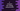# Compare two strings in C using strcoll()### Compare two strings in C using strcoll() :

In this tutorial, we will learn how to use strcoll() method in C to compare two different strings. The program will take two input strings from the user and compare them using strcoll() method. strcoll method has the following properties :

1. It takes two strings as input .
2. It compares both strings and returns one integer.
3. If fist String is less than second String, it returns one negative number. If greater than second String, returns one positive number and if equal, it returns 0.

So, mainly we will check if the return value of strcoll() is 0 or not. If 0, both strings are equal. Otherwise not. Let’s take a look into the program first :

### C program to check if two Strings are equal or not :

``````#include <stdio.h>
#include <string.h>

int main()
{
//1
char firstString;
char secondString;

//2
printf("Enter the first string : ");
fgets(firstString,100,stdin);

//3
printf("Enter the second string : ");
fgets(secondString,100,stdin);

//4
int result = strcoll(firstString,secondString);

//5
if(result == 0){
printf("Both strings are equal.\n");
}else{
printf("Strings are not equal.\n");
}

}``````

### Explanation :

1. Create two variables to store the user input string : firstString and secondString.
2. Ask the user to enter the first string and store it in firstString variable.
3. Similarly, ask the user to enter the second string and store it in secondString variable.
4. Call the function strcoll() by passing both firstString and secondString as parameter. Save the return value in integer variable result.
5. Check if result is equal to zero or not. If yes, then both strings are equal, otherwise not. Print out the message.

### Sample Output :

``````Enter the first string : First String
Enter the second string : Second String
Strings are not equal.

Enter the first string : Hello World
Enter the second string : Hello World
Both strings are equal.

Enter the first string : Hello World !!!
Enter the second string : hello world !!!
Strings are not equal.``````

Note that this comparison is case sensitive